## How do I generate a numeric Vector from a function...

My goal is to generate incremental values of a function to plot using dataplot. Why does the first example generate numeric values in the Vector and the second example does not?

fenq := f -> 2.1^(f+1):
pf := Vector(5, fenq)
[  4.41   ]
[  9.261  ]
pf := [ 19.4481 ]
[40.84101 ]
[85.766121]
fseqn := (Se, f) -> Se^(f+1):
psf := Vector(5, fsenq(2.1, j))

I can plot the first data set using dataplot(pf). I cannot plot the second data set.

## remove Elements from List based on Index...

Hi, I have been looking at various questions which are similar. But, I could not exactly what I am looking for.

https://www.mapleprimes.com/questions/99679-Removing-Elements-In-A-List

In the above link it is useful to remove elemets of specific type(identical in two lists). I am looking for removing elements from list with specific Index.

L := [1,1,a,c,1];
indexList:=[3,5]

Now based on IndexList I would like to remove the elements from L. That is I want to delete L[IndexList] and L[indexList]. I have tried to do it using

`remove(has, L, L[indexList]); `

It is working when there are no identitical elements. In my case it fails to delete exact exlemens.

`subs(seq(A[indexList[i]] = NULL, i = 1 .. nops(indexList)), A);` Both are working in same way. Not suitable when having identical elements in a list
How one could delete(remove) elements based on index??

## The subs function does not work as intended?...

Hi,

I'm very new at maple and trying to understand its engine, but it is really hard sometimes when it doesn't make sense what it is doing. I have a function that gives me

com2(e,e,f)=eh+he

and then I would like to substitute eh into <1,0> and he into <0,1>, so I write

subs({eh=<1,0>, he=<0,1>}, com2(e,e,f))

which gives me the following answer

eh+he

Here comes the confusing part. When I plug in eh+he directly into the subs thing I get

<1,1>

Why are these two outputs different from each other? To clarify, why is subs({eh=<1,0>, he=<0,1>}, com2(e,e,f))=/=subs({eh=<1,0>, he=<0,1>}, eh+he)?

## write combinations, programming...

Is there a way to program this:

I have to write an equation like this (x1+x2)*(x1+x3)*(x2+x3)=0. Left side is a combination, k=2 and n=3 so the number of terms is 3. For small number of terms this is not a problem to type, but if I have x1,x2,x3,x4,x5,x6, the number of combinations is 15 so it is complicated. Is there a way how to program something like this in Maple or I have to do it manually?

Thanks!

## Parametric plot3d...

Hello,

I have two function :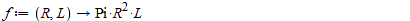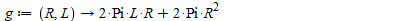And

A={g(R,L)=1}=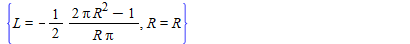(I will call that L : L0=h(R))

So I want to represent f(A) in 3D

So I tried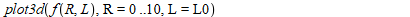It does not work, and I do not see how I could do otherwise...

## simplify an equation ...

hi.

According to the fhgure attaceh how i can gain the equation (2-27) . I write the equation (2-26) in maple but I couldnot to gain that result.

If possible to reach equation via maple?

Thanks(1)(2)## The command print placed within a while loop does ...

The command print placed within a while loop does not produce any printout. How do I  make MAPLE to produce  it?

## How to combine two plot3d animations?...

I am trying to animate two variables in a 3D plot. Basically I would like to combine these two animations into one simultaneous animation:

`animate(plot3d, [[y, x, (1/3)*Pi], x = 0 .. 2*Pi, y = 0 .. R, coords = spherical, scaling = constrained], R = 0 .. 5)`

and

`animate(plot3d, [[y, x, (1/3)*Pi], x = 0 .. p, y = 0 .. 5, coords = spherical, scaling = constrained], p = 0 .. 2*Pi)`

so that both animations start and end simultaneously. Is this possible?

## Best fitting line for discrete data ...

Hi
I appreciate your help forfinding the best fitting line for a discrete series.

Starting with 1978 as base year and counting by two's the five year overage global temperature. where

the temperature is given by the data : x,...,x

1) Find the best  fitting line : x[n+1]=a*x[n]+b
2) Assuming we can extrapolate, find the predicted value x

Fitting_best_line.mw

## intersection of inequal plot 3d ...

Hi everybody, i want to plot the intersection volume of three inequality, how can i do it?

 > restart
 > d1:=3:d2:=3:d3:=5:
 > plots:-implicitplot3d([x^2+y^2+z^2>

## Maple giving different answers. Same equation but ...

Hi,

I am trying to solve the equation below. First method was subbing in all the parameters into the equation and the second method is plugging all the parameters straight into the equation. As you can see below, they give different results. Can someone please help me? I've been scratching my head for the past hr trying to figure out what am I doing wrong.

Method 1:

Y1 := P*b*x*(-b^2+l^2-x^2)/(6*l*E*I)

maxdeflection := subs(P = 60000, b = 2050, E = 16000, I = 3062827708, l = 6600, x = (50/3)*sqrt(47229), Y1)

=-(77224295347218906250/297)*sqrt(47229)

Method 2:

Y2 := (50/3)*(60000*2050)*sqrt(47229)*(6600^2-((50/3)*sqrt(47229))^2-2050^2)/(16000*(6*6600)*3062827708)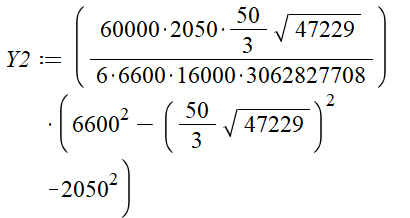=(50426796875/1819319658552)*sqrt(47229)

Thank you!

## Problem extracting roots of cubic polynomials...

Using Maple 2017.3, I ask for the roots of x3-x2-8x+8 and I get the following result:

roots(x^3-x^2-8*x+8);
[[1, 1]]

However, I know that (2*sqrt(2)) and -(2 * sqrt(2)) are also roots. Is this a bug or something I do not understand about the "roots" command?

## thismodule, abstractly indexed...

Here's a slightly reduced form of a little module from some code that I posted recently:

```KandR:= module()
local
a, b, c, e, #parameters

#procedure that lets user set parameter values:
ModuleApply:= proc({
a::algebraic:= KandR:-a, b::algebraic:= KandR:-b,
c::algebraic:= KandR:-c, e::algebraic:= KandR:-e
})
local k;
for k to _noptions do thismodule[lhs(_options[k])]:= rhs(_options[k]) od;
return
end proc
;
end module:

```

The purpose of the module is simply to be a container for the four parameters and to provide a simple ModuleApply interface by which they can be set, reset, and/or unset.

I very often use a procedure parameter of a ModuleApply to set a local variable of same name in the module. Because of the name conflict, thismodule needs to be used in these situations. I see this as the primary use of thismodule. In the module above, the purpose of the line

for k to _noptions do thismodule[lhs(_options[k])]:= rhs(_options[k]) od;

is to avoid the need to explictly use the parameters yet a third time. First off, I am amazed that this works! I've had many disappointments with thismodule (which is essentially undocumented---its miniscule help page is nearly worthless). I am using Maple 2018, release 1. Another Maple 2018 user (not sure which release) reports that the above line gives an error (when executed) that thismodule's index must be a name.

Question 1: What's up with that?

[Edit: It's been determined that the problem was due to an unfortunate global assignment in that user's initialization file rather than different behavior of thismodule. So, I consider Question 1 to be completely answered, and it should be ignored.]

Question 2: The for loop is not entirely satisfying to me. Is there a better way?

Question 3: Ideally, I'd like to explicity use the four parameters once, not two or three times. Is there a way? If I need to use a container for the parameters (such as a Record), to achieve that, I'd be happy to do that, and I wouldn't mind needing to invoke that container's name any number of times.

Note that op and exports can be applied to thismodule to extract the module's operands. I have found this occasionally useful.

Question 4: What are some other good uses for thismodule? The one and only example given on its help page seems ridiculous to me.

The docs say that you can assign initial values to a record as shown in this screenshot:I would expect the last two lines of output to be 1, 2. The slighly more complicated example in the docs does not work as expected either. This is the worksheet: queery.mw

I can assign to a record subsequently, but that makes for very prolix code,

Thanks for any help.

## string to number conversion from TextArea...

How do we use the command convert(expression, number format) in the conversion of number input in a textarea?radianToDegree.mwI want conversion from string to float and evaluate radian to degree and show it in textareaDegree.

I am unable to do the operation. 6 radian = 6x180/2 (pi) degrees must be shown degree textbox.

Thanks.

Ramki